# Test: Name Reaction Level - 1

## 30 Questions MCQ Test Organic Chemistry | Test: Name Reaction Level - 1

Description
Attempt Test: Name Reaction Level - 1 | 30 questions in 60 minutes | Mock test for Chemistry preparation | Free important questions MCQ to study Organic Chemistry for Chemistry Exam | Download free PDF with solutions
QUESTION: 1

Solution:
QUESTION: 2

Solution:
QUESTION: 3

### The major product formed in the following reaction is: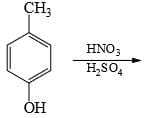Solution:
QUESTION: 4

Choose the reaction sequence that could be used to perform the following transformation: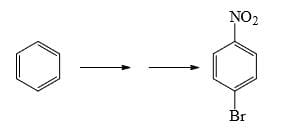Solution:
QUESTION: 5

What could be reagents A and B for the following reactions: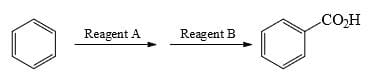Solution:
QUESTION: 6

What could be the reagent to complete the following reaction: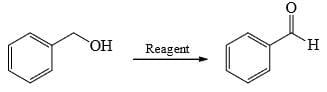Solution:
QUESTION: 7

Choose order that has the following compounds correctly arranged with respect to increasing rate of reaction with LiAlH4 (most reactive compound on the right).

Solution:
QUESTION: 8

Choose the reaction(s) that will not proceed as shown hereunder:

Solution:

Reduction of amide by LAH gives amine but hydrolysis of amine not give alcohol.

QUESTION: 9

Predict the product of the following aldol condensation: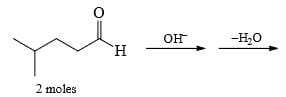Solution:
QUESTION: 10

Which of the following structure is more stable:

Solution:

The negative charge is delocalized and NO2 shows -I and -M effect and hence stabilizes -ve charge.

QUESTION: 11

Which of the following is most reactive towards aqueous HBr:

Solution:
QUESTION: 12

Ethylbenzene when treated with chlorine in presence of light mainly gives:

Solution:
QUESTION: 13

The following alcohol is treated with Conc. H2SO4, the major product obtained is: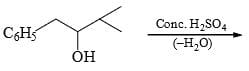Solution:
QUESTION: 14

The major product formed in the following reaction is: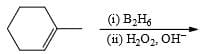Solution:
QUESTION: 15

Give the nature of And B in the given reaction: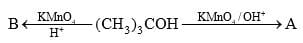Solution:
QUESTION: 16

The reaction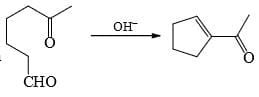is an example of:

Solution:
QUESTION: 17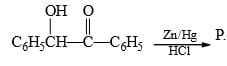Here, P should be:

Solution:
QUESTION: 18

Nitrobenzene can be reduced to aniline by:

(I) H2/Ni

(II) Sn/HCl

(III) Zn/NaOH

(IV) LiAlH4

Solution:

Explanation : When nitrobenzene is reduced with a metal and acid (Sn/HCl, Zn/HCl etc.), aniline is obtained. The intermediate products nitrosobenzene and phenylhydroxylamine are never isolated because these are reduced more rapidly than nitrobenzene.

However, the catalytic hydrogenation of nitrobenzene gives aniline.

PhNO2  (H2+Ni)------------->PhNH2

​

Azobenzene is formed when nitrobenzene is reduced by LiAlH4.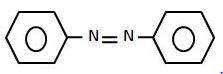QUESTION: 19

1-Methylcyclopentene can be converted into 2-methylcyclopentanol by:

Solution:
QUESTION: 20

2-Methylpropanol-2 can be obtained by the acid-catalyzed hydration of:

Solution:
QUESTION: 21

A new carbon-carbon bond formation is possible in

Solution:
QUESTION: 22

The major product formed in the following reaction is: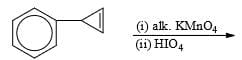Solution:
QUESTION: 23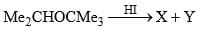Predict the nature of product and the type of reaction involved in their formation.

Solution: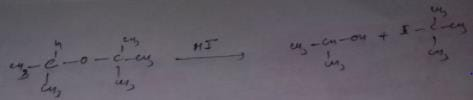QUESTION: 24

Anisole is treated with HI under two different conditions: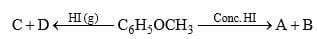The nature of A and D will be

Solution:
QUESTION: 25

The ethereal linkage (—C—O—C—) is cleaved by:

Solution:
QUESTION: 26

Predict the compounds A and B in the following reactions: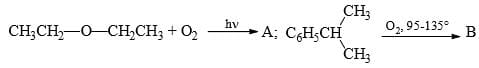Solution:

Option C correct. Due to Photochemical conditions O-O bond of Oxygen will be cleaved.

QUESTION: 27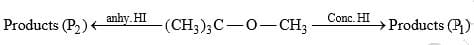The products P1 and P2 respectively are:

Solution: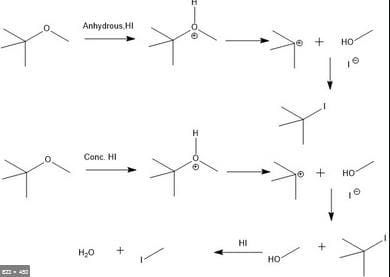QUESTION: 28

Which of the following statement is true regarding amount of AlCl3 required during Friedel-Craft acetylation using acetyl chloride or acetic anhydride:

Solution:
QUESTION: 29

Which of the following gives effervescences of CO2 with NaHCO3 solution:

Solution:
QUESTION: 30

2,4,6-Trinitrophenol can be prepared in good yield:

Solution:Use Code STAYHOME200 and get INR 200 additional OFF Use Coupon Code